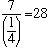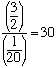Subject: Dividing Fractions Name: Paula Who is asking: Student Level: Middle Question: Why do you have to change the division sign to a multiplication sign and invert the fraction that follows the division sign in order to get the answer to a division problem when you're working with fractions? Hi Paula, One technique that may help you understand is to think in terms of money. For example the question How many 5 dollar bills are there in 200 dollars? has the answer 200/5 = 40. In a similar way the question How many quarters are there in 7 dollars? has the answerOr, if you have a dollar and a half (3/2 dollars) and you want to know how many nickles there are in your dollar and a half then, since a nickle is one-twentieth of a dollar, the answer isIf you want a more algebraic answer you can find one in Invert and multiply the answer to a similar question we received some time ago. Cheers, Penny Go to Math Central